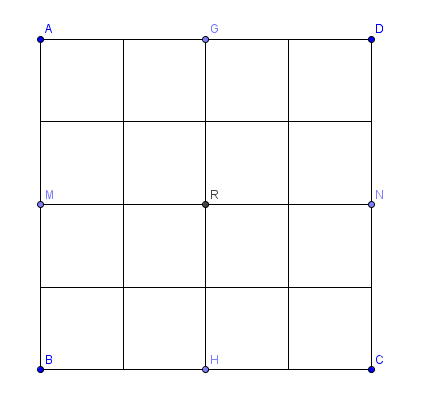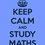# RMO 2014 Delhi Region Q.4Is it possible to write the numbers $$17,18,19,\ldots ,32$$ in a $$4\times 4$$ grid on unit squares, with one number in each square, such that the product of the numbers in each $$2\times 2$$ sub-grids $$AMRG, GRND, MBHR$$ and $$RHCN$$ is divisible by 16?

• You can find rest of the problems here

• You can find the solutions hereNote by Aneesh Kundu
6 years, 6 months ago

This discussion board is a place to discuss our Daily Challenges and the math and science related to those challenges. Explanations are more than just a solution — they should explain the steps and thinking strategies that you used to obtain the solution. Comments should further the discussion of math and science.

When posting on Brilliant:

• Use the emojis to react to an explanation, whether you're congratulating a job well done , or just really confused .
• Ask specific questions about the challenge or the steps in somebody's explanation. Well-posed questions can add a lot to the discussion, but posting "I don't understand!" doesn't help anyone.
• Try to contribute something new to the discussion, whether it is an extension, generalization or other idea related to the challenge.
• Stay on topic — we're all here to learn more about math and science, not to hear about your favorite get-rich-quick scheme or current world events.

MarkdownAppears as
*italics* or _italics_ italics
**bold** or __bold__ bold
- bulleted- list
• bulleted
• list
1. numbered2. list
1. numbered
2. list
Note: you must add a full line of space before and after lists for them to show up correctly
paragraph 1paragraph 2

paragraph 1

paragraph 2

[example link](https://brilliant.org)example link
> This is a quote
This is a quote
    # I indented these lines
# 4 spaces, and now they show
# up as a code block.

print "hello world"
# I indented these lines
# 4 spaces, and now they show
# up as a code block.

print "hello world"
MathAppears as
Remember to wrap math in $$ ... $$ or $ ... $ to ensure proper formatting.
2 \times 3 $2 \times 3$
2^{34} $2^{34}$
a_{i-1} $a_{i-1}$
\frac{2}{3} $\frac{2}{3}$
\sqrt{2} $\sqrt{2}$
\sum_{i=1}^3 $\sum_{i=1}^3$
\sin \theta $\sin \theta$
\boxed{123} $\boxed{123}$

## Comments

Sort by:

Top Newest

The answer is - impossible. Let us multiply all the numbers from 17 to 32 (the product will be equal to the product of four results for the sub-grids). It can easily be shown that the highest power of 2 in the product be 16. But as the number 32 (=2^5) should get in one of the sub-grids, the resultant 11th power of 2 should be distributed among three other grids. But it means that at least one of the products of the last three grids cann't be divisible by the fourth power of 2 equal to 16.

- 6 years, 6 months ago

Log in to reply

yes , $2^{4} =16$

First let us write the prime factorization of all the even numbers ,

$18 = 2 * 9$

$20 = 2^{2} * 5$

$22 = 2 * 11$

$24 = 2^{3} * 11$

$26 = 2 * 13$

$28 = 2^{2} * 7$

$30 = 2 * 15$

$32 = 2^{5}$

The product of the numbers in 2x2 square to be divisible by 16 , each square should contain at least 2 raise to the power 4 . thus if in one 2x2 square if only 32 and other odd numbers is there - its satisfys . similarly - (18 , 20 ,22) , (20 ,28) ,(18 , 22 , 26 ,30) etc

- 6 years, 6 months ago

Log in to reply

Nope If u place 32 in one of the sub grids u still need 12 factors of 2 (to be placed in the 3 other sub grids)but we are left with only 11 factors so such an arrangement is not possible

- 6 years, 6 months ago

Log in to reply

Yes, I tried combinations, but all fell in vain.

- 6 years, 6 months ago

Log in to reply

Oh yes sorry i used 18 2 times Thank you . How much you are expecting to get right?@Aneesh Kundu

- 6 years, 6 months ago

Log in to reply

I solved 4

Don't know how many are correct

- 6 years, 6 months ago

Log in to reply

We have $18=2\times9$, $20=2^{2}\times5$, $22=2\times11$, $24=2^{3}\times3$, $26=2\times13$,$28=2^{2}\times7$, $30=2\times15$, $32=2^5$.

I will consider the set of powers of 2,$A$= ${1,2,1,3,1,2,1,5,0,0,0,0,0,0,0,0}$ ($0$ corrsponds to the power of $2$ in the odd numbers).

Suppose we can partition the set $A$ into $4$ subsets $X_{1},X_{2},X_{3},X_{4}$ where $|X_{i}|=4$ where $i=1,..,4$ such that $k_{i}≥4$ where $k_{i}$ denotes the sum of the elements of $X_{i}$.

Note that the sum of the elements of $A$ is $16$.

Clearly ${5}$ belongs to one of the $4$ subsets,say $X_{1}$.Then $k_{1}≥5$

Hence,$k_{2}+k_{3}+k_{4}=16-k_{1}≤11$ contradiction!(since sum of them is $≥12$)

So, the arrangement given in the problem is impossible.

- 6 years, 6 months ago

Log in to reply

it would have been possible if we had one number having 2^1 . by the way .. i got problems 1 ,4 right . it was my first experience in rmo . . .

- 6 years, 6 months ago

Log in to reply

I solved it using pigeon hole principle

- 6 years, 5 months ago

Log in to reply

×

Problem Loading...

Note Loading...

Set Loading...